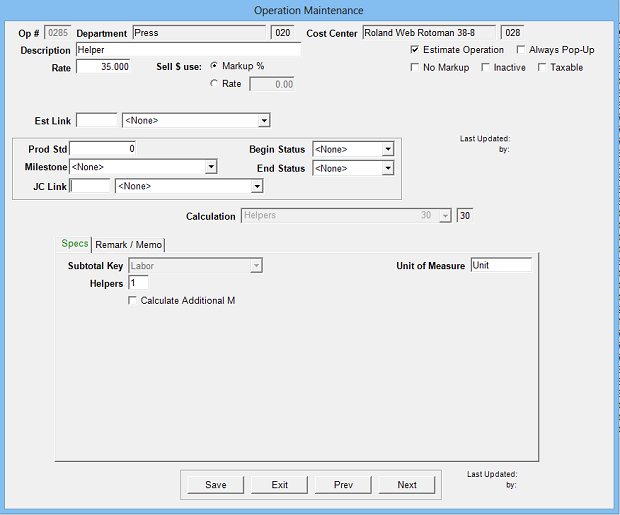Estimates the cost of helpers to a labor operation using an hourly rate and the calculated hours from the preceding labor operation.

Operation Setup Requirements:
1. Description
2. Rate: Cost per hour (per helper)
3. Sell \$ use: Markup % (recommended) or alternate sell rate
4. Calc: 30
5. Sub Key: Labor
6. Helpers: Default number of helpersEstimate Specification Input Prompts:

1. Helpers
2. J%
3. Remarks
4. MemoCalculation:

Hours From preceding labor operation x J% = Estimated Hours

Helpers x Estimated Hours x Rate = Cost \$

Cost \$ x Markup % = Markup \$

Cost \$ + Markup \$ = Sell \$